## Mike on MATLAB GraphicsGraphics & Data Visualization

Note

Mike on MATLAB Graphics has been archived and will not be updated.

# Pretriangulation

### Pretriangulation

An interesting question went by on MATLAB Answers the other day. I've simplified it a bit here, but it looked something like this:

rng(0)
cla
nfaces = 5000;
nsides = 6;
nframes = 35;

ang = 0:(nsides-1) * pi * 2 / nsides;
x = repmat(cos(ang)',[1 nfaces]);
y = repmat(sin(ang)',[1 nfaces]);
z = repmat([1:nfaces],[nsides 1]);
c = rand(1,nfaces);
xoff = repmat(randn(1,nfaces),[nsides, 1]);
yoff = repmat(randn(1,nfaces),[nsides, 1]);
h = patch(x+xoff,y+yoff,z,c);
h.FaceColor = 'flat';
h.EdgeColor = 'none';
axis equal
xlim manual
ylim manual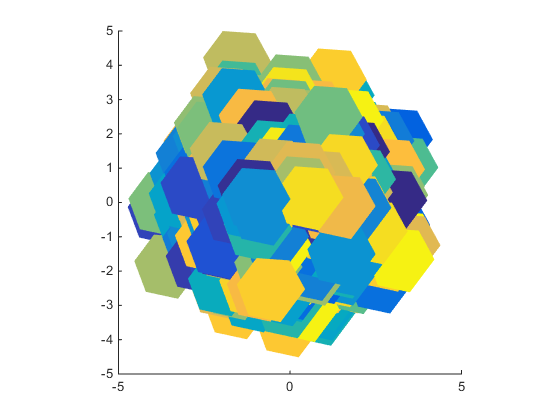That creates a patch object which draws a bunch of filled hexagons. Now we want to move them around. I'm going to use these random rotation angles to spin them around the origin at various speeds.

angstep = rand(1,nfaces);
ca = cos(angstep);
sa = sin(angstep);
ca = repmat(ca,[nsides 1]);
sa = repmat(sa,[nsides 1]);
ca = ca(:);
sa = sa(:);


We can do that by modifying the Vertices property.

tic
for i=1:nframes
x = h.Vertices(:,1);
y = h.Vertices(:,2);
h.Vertices(:,1) =  ca.*x + sa.*y;
h.Vertices(:,2) = -sa.*x + ca.*y;
drawnow;
end
disp([num2str(nframes/toc) ' frames per second'])

3.3139 frames per second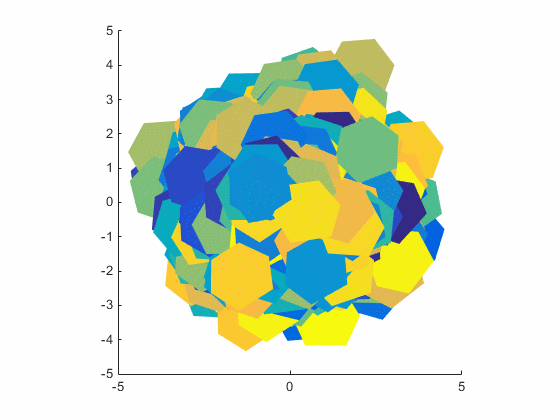That's really slow, isn't it? What can we do to improve this?

There are a number of approaches we could take to speed this up. One which comes to mind is to use hgtransform like we did a couple of weeks ago. We could try that, but it actually might not be as useful here because each of those hexagons is so much simpler than the blobs we were drawing in that example. Sending the graphics card a 4x4 transform matrix instead of one of those blobs was a big win, but sending a 4x4 instead of a little hexagon wouldn't be as big a win.

So lets look at a different technique. This technique is one we call "pretriangulation". The basic idea here is that a big part of the problem is that the patch object has hexagons, but the graphics card only knows how to draw triangles. Every time we change the Vertices, the patch object is converting the hexagons into triangles. This step is known as polygon triangulation. Good name, huh? Triangulation is actually one of the most interesting corners of computer graphics and compuational geometry. We'll be visiting it many times in future blog posts.

First we need to get an idea about how hard triangulation is. It doesn't seem like splitting a hexagon into triangles is a hard problem.

fig2 = figure;
ang = (0:5) * pi * 2 / 6;
x = cos(ang');
y = sin(ang');
p = patch('Vertices',[x, y, zeros(size(ang'))],'Faces',[1:6]);
p.FaceColor = 'yellow';
axis equal

r = 7/8;
for i=1:6
text(r*cos(ang(i)),r*sin(ang(i)),['Pt_' num2str(i)],'HorizontalAlignment','center');
end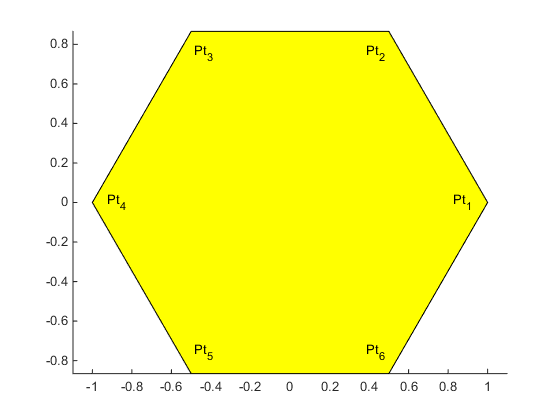We can just slice it like so:

line(x([1 3 5 1]),y([1 3 5 1]),'Color','black');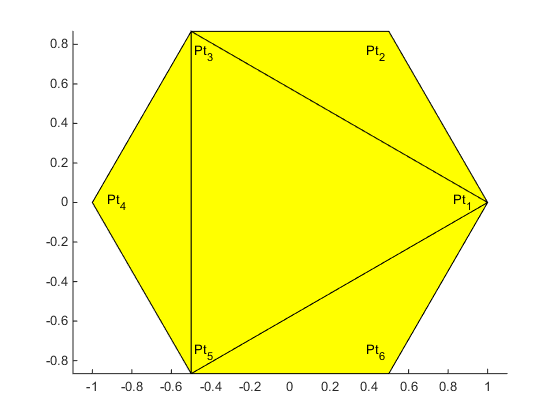But patch needs to worry about lots of different types of polygons. It can tell that we're dealing with hexagons because of the size of the Faces property, but hexagons come in a lot of different shapes. Let's take a quick look at some.

cla
for r=1:3
for c=1:5
p = patch('Vertices',[3*c+randn(6,1),3*r+randn(6,1),zeros(6,1)],'Faces',1:6);
p.FaceColor = rand(1,3);
end
end
axis tight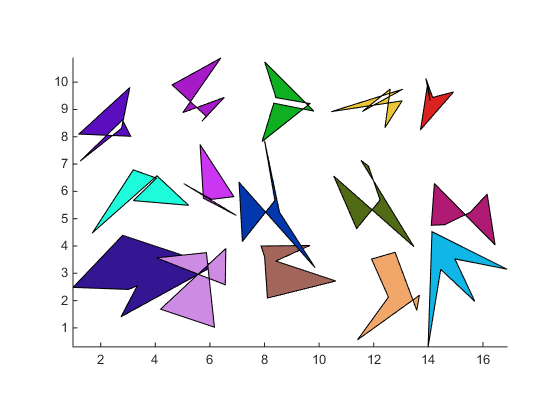Hmm, maybe it's not as simple as it seems. It turns out that writing a really robust triangulator is pretty challenging, and it's very hard to write a robust one which is also very fast. This means that if we care about performance, we probably want to avoid triangulation whenever we can.

It turns out that in our example above we actually only need to triangulate once. That's because because that rotation we're doing is what's called an affine transformation. That means that the shape of the objects being transformed doesn't change. And that means that the triangulation can be reused.

The problem is that the patch object can't really figure that out. It just knows that the Vertices property has changed. Figuring out whether the old triangulation would still work turns out to be almost as expensive as just redoing the triangulation.

But in this case the triangulation is actually simple enough that we can do it ourselves. We can just follow the diagram above to turn each hexagon into four triangles. That will conver the 5,000 by 6 Faces array which represents 5,000 hexagons into a 20,000 by 3 array which represents 20,000 triangles. When we give patch a Faces array with 3 columns, it will know that it can send them straight over to the graphics card without doing any triangulation.

close(fig2);

f = h.Faces;
f2 = zeros(4*nfaces,3);

% First the big triangle in the center
f2(1:4:end,1:3) = f(:,[1,3,5]);

% Then the three little triangles around the perimeter
f2(2:4:end,1:3) = f(:,[1,2,3]);
f2(3:4:end,1:3) = f(:,[3,4,5]);
f2(4:4:end,1:3) = f(:,[5,6,1]);

h.Faces = f2;

% Expand the colors by 4 too
c = h.FaceVertexCData;
c2 = repmat(c',[4 1]);
c2 = c2(:);
h.FaceVertexCData = c2;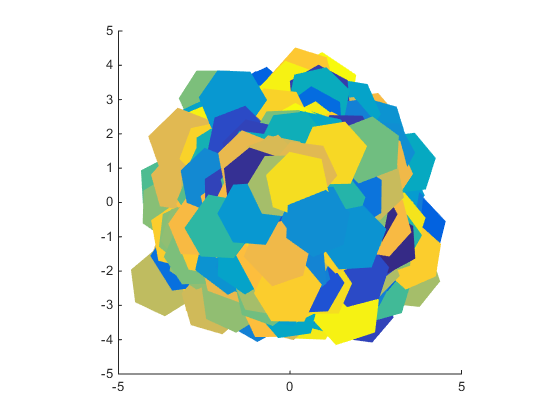So let's try moving these pretriangulated hexagons around the same way we did before.

tic
for i=1:nframes
x = h.Vertices(:,1);
y = h.Vertices(:,2);
h.Vertices(:,1) =  ca.*x + sa.*y;
h.Vertices(:,2) = -sa.*x + ca.*y;
drawnow;
end
disp([num2str(nframes/toc) ' frames per second'])

34.1455 frames per second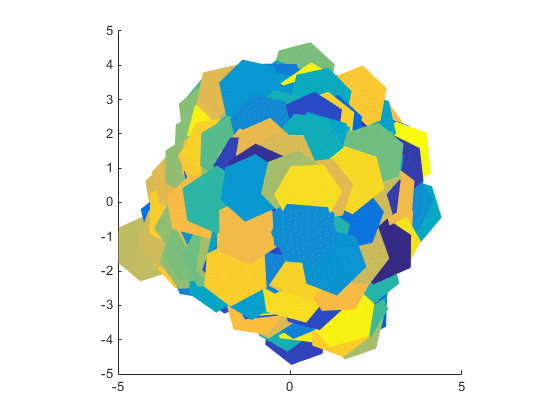That's about 10 times as fast, which is pretty good. And it gets us over that 24 frames per second barrier that we want to cross for smooth animations.

But this approach does have some limitations. If we had been doing something to the hexagons which changed their shapes, then we couldn't reuse the original triangulation. And we were lucky that the triangulation was so simple in this case. For more complex polygons it can get pretty tricky. We can use the triangulator which patch uses, but it's kind of tricky to use. It'd be really neat if we could just ask patch to do the pretriangulation for us, wouldn't it? Also, if we hadn't turned the edges off then we would be seeing the interior edges between the triangles.

But even with those limitations, you may find cases where this is a useful technique to have in your MATLAB graphics bag of tricks.

Published with MATLAB® R2014b